# Cross Product

A vector has magnitude (how long it is) and direction:Two vectors can be multiplied using the "Cross Product" (also see Dot Product)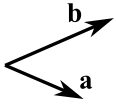The Cross Product a × b of two vectors is another vector that is at right angles to both: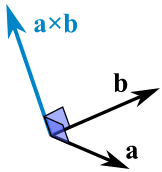And it all happens in 3 dimensions!

The magnitude (length) of the cross product equals the area of a parallelogram with vectors a and b for sides:See how it changes for different angles:

The cross product (blue) is:

• zero in length when vectors a and b point in the same, or opposite, direction
• reaches maximum length when vectors a and b are at right angles

And it can point one way or the other!

So how do we calculate it?

## Calculating

#### We can calculate the Cross Product this way: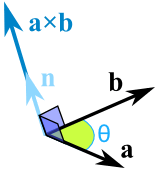a × b = |a| |b| sin(θ) n

• |a| is the magnitude (length) of vector a
• |b| is the magnitude (length) of vector b
• θ is the angle between a and b
• n is the unit vector at right angles to both a and b

So the length is: the length of a times the length of b times the sine of the angle between a and b,

Then we multiply by the vector n so it heads in the correct direction (at right angles to both a and b).

#### OR we can calculate it this way: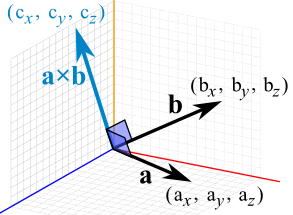When a and b start at the origin point (0,0,0), the Cross Product will end at:

• cx = aybz − azby
• cy = azbx − axbz
• cz = axby − aybx

### Example: The cross product of a = (2,3,4) and b = (5,6,7)

• cx = aybz − azby = 3×7 − 4×6 = −3
• cy = azbx − axbz = 4×5 − 2×7 = 6
• cz = axby − aybx = 2×6 − 3×5 = −3

Answer: a × b = (−3,6,−3)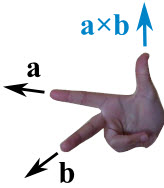## Which Direction?

The cross product could point in the completely opposite direction and still be at right angles to the two other vectors, so we have the:

"Right Hand Rule"

With your right-hand, point your index finger along vector a, and point your middle finger along vector b: the cross product goes in the direction of your thumb.

## Dot Product

The Cross Product gives a vector answer, and is sometimes called the vector product.

But there is also the Dot Product which gives a scalar (ordinary number) answer, and is sometimes called the scalar product.Question: What do you get when you cross an elephant with a banana?